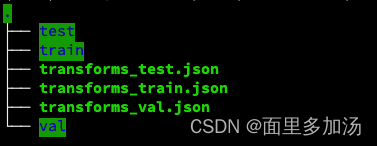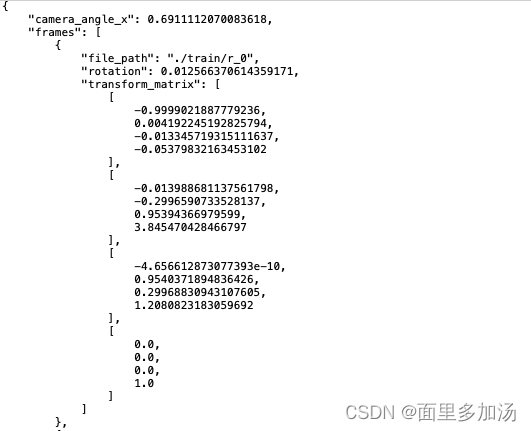0

# NeRF 源码分析解读（一）

## 前言

NeRF 是三维视觉中新视图合成任务的启示性工作，最近领域内出现了许多基于 NeRF 的变种工作。本文以pytorch 版 NeRF 作为基础对 NeRF 的代码进行分析。

1. 数据的加载
2. 光线的生成
3. NeRF 网络架构
4. 渲染过程

## 一、数据的加载train、test、val 三个文件夹下包含了训练要用到的 .png 图像，每个文件夹下包含 100 个文件。.json 文件包含了相机的 camera2word 转置矩阵，下图展示了部分文件中的内容。关于此转置矩阵不再展开叙述，具体知识可查看 SLAM 14 讲。了解以上基本信息后解析数据加载的代码。frame 的值是一个列表，其中列表中的值是字典

``````deftrain():# 设置参数
parser = config_parser()
args = parser.parse_args()...elif args.dataset_type =='blender':
i_train, i_val, i_test = i_split

# 设定边界框的远近边界
near =2.
far =6.# 将 RGBA 转换成 RGB 图像if args.white_bkgd:# 如果使用白色背景
images = images[...,:3]*images[...,-1:]+(1.-images[...,-1:])else:
images = images[...,:3]
``````

``````defload_blender_data(basedir, half_res=False, testskip=1):"""
:param basedir: 数据文件夹路径
:param half_res: 是否对图像进行半裁剪
:param testskip: 挑选测试数据集的跳跃步长
:return:
"""
splits =['train','val','test']
metas ={}for s in splits:# 分别加载三个 .json 文件，保存到字典中withopen(os.path.join(basedir,'transforms_{}.json'.format(s)),'r')as fp:

all_imgs =[]
all_poses =[]
counts =for s in splits:
meta = metas[s]# 加载 .json 文件中的内容
imgs =[]
poses =[]# 如果是 train 文件夹，连续读取图像数据if s=='train'or testskip==0:
skip =1else:
skip = testskip

for frame in meta['frames'][::skip]:# 以指定步长读取列表中的字典
fname = os.path.join(basedir, frame['file_path']+'.png')

poses.append(np.array(frame['transform_matrix']))# 读取转置矩阵
imgs =(np.array(imgs)/255.).astype(np.float32)# 此时 图像数组是一个 [n, H, W, 4] 的数组
poses = np.array(poses).astype(np.float32)# [n, 4, 4]
counts.append(counts[-1]+ imgs.shape)# 用于标定到train、test、val

all_imgs.append(imgs)# 包含了 train、test、val 的图像的列表
all_poses.append(poses)

i_split =[np.arange(counts[i], counts[i+1])for i inrange(3)]

imgs = np.concatenate(all_imgs,0)# 把列表聚合称为一个数组 [N, H, W, 4]
poses = np.concatenate(all_poses,0)

H, W = imgs.shape[:2]
camera_angle_x =float(meta['camera_angle_x'])
focal =.5* W / np.tan(.5* camera_angle_x)# 计算焦距# 制作用于测试训练效果的 渲染pose
render_poses = torch.stack([pose_spherical(angle,-30.0,4.0)for angle in np.linspace(-180,180,40+1)[:-1]],0)# 为了节省内存开销可以选择只加载一半的数据，这里不再展开解释if half_res:
H = H//2
W = W//2
focal = focal/2.

imgs_half_res = np.zeros((imgs.shape, H, W,4))for i, img inenumerate(imgs):
imgs_half_res[i]= cv2.resize(img,(W, H), interpolation=cv2.INTER_AREA)
imgs = imgs_half_res
# imgs = tf.image.resize_area(imgs, [400, 400]).numpy()return imgs, poses, render_poses,[H, W, focal], i_split
``````

``````imgs : 根据 .json 文件加载到的所有图像数据。（N，H，W，4）N 代表用于 train、test、val 的总数量
poses : 转置矩阵。（N，4，4）
render_poses : 用于测试的 pose 。（40，4，4）
i_split :[[0:train], [train:val], [val:test]]
``````

NeRF源码分析解读（二）

### “NeRF 源码分析解读（一）”的评论:

##### 关于作者##### overfit同步小助手